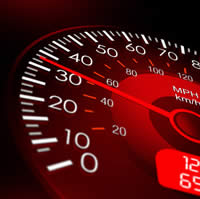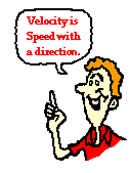# Difference between Speed and Velocity

##### Key Difference: Speed is the rate at which an object covers a distance. Velocity not only determines the speed of the object in motion but also the direction of the object in motion.

In everyday usage, the terms speed and velocity is often used interchangeably and it does not cause that much of a problem. However, when it comes to science and mathematics, these two concepts are different from each other.In kinematics, speed is the rate at which an object covers a distance. It is a scalar quantity. Scalar means that it is used to measure the magnitude or strength of something. It has to do much with just trying to learn how much. Speed is measured using meters, kilometer, and miles per hour/per second. For air and marine travel, it is measured in knots per hour. Italian physicist Galileo Galilei is credited as being the first person to measure speed by considering the distance covered by an object. Galileo defined speed as the distance covered per unit of time.

There are two ways to measure speed: average speed or instantaneous speed. Average speed is the total distance covered over the time interval. For example: If a car covers the distance of 320 kilometers over a period of 4 hours, then the average speed would be 320km divided by 4hr, which would result in the speed being 80 km per hour. Average speed does not describe the speed variations that may have taken place during the trip such as traffic stops, reduction or acceleration in speed. Instantaneous speed is the speed that is shown on the speedometer at any given moment. If while driving, the driver looks at the speedometer, the instantaneous speed would be the speed on the meter. If a person continues to drive at that one speed for an hour, (i.e. 50 km) the speed would be considered as 50km/hr.Velocity is the rate of change of position of an object, equivalent to a specification of its speed and direction of motion. It is a vector quantity. Velocity not only determines the speed of the object in motion but also the direction of the object in motion. In order to maintain a constant velocity, an object must travel in a certain direction for a certain amount of time. If the object changes direction, the velocity of the object also changes. The path must also not be circular or curve. An object which travels in a circle and comes back to its starting point is said to have zero velocity. For example, a car that is travelling on a circular circuit at 90 km/hr is said to have a speed of 90 km/hr, however it does not have a velocity.

Vector quantity is when an object requires both magnitude as well as direction in order to define it. The scalar absolute value (magnitude) of velocity is known as speed. So a velocity without a direction or heading is basically the speed. Another example where a car is travelling 70 km/hr in the direction north is said to have a velocity of 70 km/hr, north. Velocity is commonly used to determine the object’s speed and the direction of travel change at each point in time. The change in rate of velocity as a function of time is known as acceleration, while ‘deceleration’ is known as ‘negative acceleration’ and written with a (-) sign. Displacement plays a huge part in determine the velocity of an object. Displacement is the shortest distance from the initial to the final position of another point.

 Speed Velocity Type of quantity Scalar quantity Vector quantity Definition Speed is the rate at which an object covers a distance. Velocity is the rate of change of position of an object, equivalent to a specification of its speed and direction of motion. Change of Direction The object can change direction and the average speed will continue to count. The object must follow one direction, if the direction of an object changes so do the velocity. Examples A car travelling at 50 km/hr would go from 0 km/hr to 30 km/hr, before reaching 50 km/her and could even cross 60. However, the average speed will be counted as the speed of the car. A car going in a straight line towards a particular direction is considered to have a velocity. If the car is going towards north and has an average speed of 30 km/hr, the velocity would be 30 km/hr, north. Equation v = d/t; where v is the average speed, d is the distance travelled and t is time it took to travel that distance.= ∆x/∆t; whereis the average velocity, ∆x is the displacement and ∆t is the time of arrival.

Image Courtesy: consumers.ofcom.org.uk, physicsclassroom.com